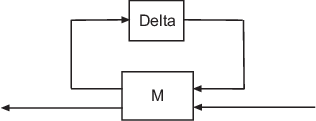# lftdata

Decompose uncertain objects into fixed certain and normalized uncertain parts

## Syntax

```[M,Delta] = lftdata(A);
[M,Delta] = lftdata(A,List);
[M,Delta,Blkstruct] = lftdata(A);
[M,Delta,Blkstruct,Normunc] = lftdata(A);
```

## Description

`lftdata ` decomposes an uncertain object into a fixed certain part and a normalized uncertain part. `lftdata` can also partially decompose an uncertain object into an uncertain part and a normalized uncertain part. Uncertain objects (`umat`, `ufrd`, `uss`) are represented as certain (i.e., not-uncertain) objects in feedback with block-diagonal concatenations of uncertain elements.

`[M,Delta] = lftdata(A)` separates the uncertain object `A` into a certain object `M` and a normalized uncertain matrix `Delta` such that `A` is equal to `lft(Delta,M)`, as shown below.If `A` is a `umat`, then `M` will be `double`; if `A` is a `uss`, then `M` will be `ss`; if `A` is a `ufrd`, then `M` will be `frd`. In all cases, `Delta` is a `umat`.

`[M,Delta] = lftdata(A,List)` separates the uncertain object `A` into an uncertain object `M`, in feedback with a normalized uncertain matrix `Delta`. `List` is a cell (or char) array of names of uncertain elements of `A` that make up `Delta`. All other uncertainty in `A` remains in `M`.

`lftdata(A,fieldnames(A.Uncertainty))` is the same as `lftdata(A)`.

`[M,DELTA,BLKSTRUCT] = lftdata(A)` returns an N-by-1 structure array `BLKSTRUCT`, where `BLKSTRUCT(i)` describes the `i`-th normalized uncertain element. This uncertainty description can be passed directly to the low-level structured singular value analysis function `mussv`.

`[M,DELTA,BLKSTRUCT,NORMUNC] = lftdata(A)` returns the cell array `NORMUNC` of normalized uncertain elements. Each normalized element has `'Normalized'` appended to its original name to avoid confusion. Note that `lft(blkdiag(NORMUNC{:}),M)` is equivalent to `A`. The normalizations for each type of uncertain element are described in Decomposing Uncertain Objects.

## Examples

collapse all

Create an uncertain matrix `A` with uncertain parameters `p1`, and `p2`. Decompose `A` into its certain part, `M`, and normalized uncertain part, `Delta`.

```p1 = ureal('p1',-3,'perc',40); p2 = ucomplex('p2',2); A = [p1 p1+p2;1 p2]; [M,Delta] = lftdata(A); ```

You can inspect the difference between the original uncertain matrix, `A`, and the result formed by combining the two results from the decomposition.

`simplify(A-lft(Delta,M)) `
```ans = 2×2 0 0 0 0 ```
`M`
```M = 4×4 0 0 1.0954 1.0954 0 0 0 1.0000 1.0954 1.0000 -3.0000 -1.0000 0 1.0000 1.0000 2.0000 ```

You can check the worst-case norm of the uncertain part using `wcnorm`. Compare samples of the uncertain part `A` with the uncertain matrix `A`.

`wcn = wcnorm(Delta) `
```wcn = struct with fields: LowerBound: 0 UpperBound: 1.0012 ```
`usample(Delta,3) `
```ans = ans(:,:,1) = 0.6294 + 0.0000i 0.0000 + 0.0000i 0.0000 + 0.0000i 0.8776 - 0.4413i ans(:,:,2) = 0.8116 + 0.0000i 0.0000 + 0.0000i 0.0000 + 0.0000i 0.2704 + 0.2907i ans(:,:,3) = -0.7460 + 0.0000i 0.0000 + 0.0000i 0.0000 + 0.0000i -0.3382 + 0.9253i ```

Create an uncertain matrix `A` with 2 uncertain real parameters `v1` and `v2`. The create an uncertain system `G` using `A` as the dynamic matrix, and numeric matrices for the input and output.

```A = [ureal('p1',-3,'perc',40) 1;1 ureal('p2',-2)]; sys = ss(A,[1;0],[0 1],0); sys.InputGroup.ActualIn = 1; sys.OutputGroup.ActualOut = 1; ```

You can decompose `G` into a certain system, `Msys`, and a normalized uncertain matrix, `Delta`. You can see from `Msys` that it is certain, and that the input and output groups have been adjusted.

```[Msys,Delta] = lftdata(sys); Msys ```
```Msys = A = x1 x2 x1 -3 1 x2 1 -2 B = u1 u2 u3 x1 1.095 0 1 x2 0 1 0 C = x1 x2 y1 1.095 0 y2 0 1 y3 0 1 D = u1 u2 u3 y1 0 0 0 y2 0 0 0 y3 0 0 0 Input groups: Name Channels ActualIn 3 Output groups: Name Channels ActualOut 3 Continuous-time state-space model. ```

You can compute the norm on samples of the difference between the original uncertain matrix and the result formed by combining `Msys` and `Delta`.

`norm(usample(sys-lft(Delta,Msys),'p1',4,'p2',3),'inf') `
```ans = 4×3 0 0 0 0 0 0 0 0 0 0 0 0 ```

Create an uncertain matrix `A` and derive an uncertain matrix `B` using an implicit-to-explicit conversion, `imp2exp`. Note that `B` has 2 uncertain parameters `R` and `K`. You can decompose `B` into certain part, `M`, and normalized uncertain part, `Delta`.

```R = ureal('R',1,'Percentage',[-10 40]); K = ureal('K',2e-3,'Percentage',[-30 30]); A = [1 -R 0 -K;0 -K 1 0]; Yidx = [1 3]; Uidx = [4 2]; B = imp2exp(A,Yidx,Uidx); [M,Delta] = lftdata(B); ```

The same operation can be performed by defining the uncertain parameters, `K` and `R`, to be extracted.

```[MK,DeltaR] = lftdata(B,'R'); MK ```
```MK = Uncertain matrix with 3 rows and 3 columns. The uncertainty consists of the following blocks: K: Uncertain real, nominal = 0.002, variability = [-30,30]%, 2 occurrences Type "MK.NominalValue" to see the nominal value, "get(MK)" to see all properties, and "MK.Uncertainty" to interact with the uncertain elements. ```
```[MR,DeltaK] = lftdata(B,'K'); MR ```
```MR = Uncertain matrix with 4 rows and 4 columns. The uncertainty consists of the following blocks: R: Uncertain real, nominal = 1, variability = [-10,40]%, 1 occurrences Type "MR.NominalValue" to see the nominal value, "get(MR)" to see all properties, and "MR.Uncertainty" to interact with the uncertain elements. ```
`simplify(B-lft(Delta,M)) `
```ans = 2×2 0 0 0 0 ```
`simplify(B-lft(DeltaR,MK)) `
```ans = 2×2 0 0 0 0 ```
`simplify(B-lft(DeltaK,MR)) `
```ans = 2×2 0 0 0 0 ```

Examine the result formed by combining the two results from the decomposition.

```[Mall,Deltaall] = lftdata(B,{'K';'R'}); Mall-M```
```ans = 5×5 0 0 0 0 0 0 0 0 0 0 0 0 0 0 0 0 0 0 0 0 0 0 0 0 0 ```

## Version History

Introduced before R2006a